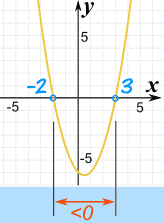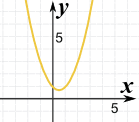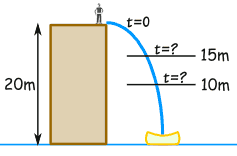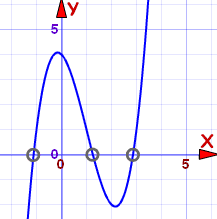... and more ...

A Quadratic Equation (in Standard Form) looks like:A Quadratic Equation in Standard Form
(a, b, and c can have any value, except that a can't be 0.)

The above is an equation (=) but sometimes we need to solve inequalities like these:

Symbol

Words

Example

>

greater than

x2 + 3x > 2
<

less than

7x2 < 28

greater than or equal to

5 ≥ x2 − x

less than or equal to

2y2 + 1 ≤ 7y

## Solving

Solving inequalities is very like solving equations ... we do most of the same things.

 When solving equations we try to find points, such as the ones marked "=0"But when solving inequalities we try to find interval(s), such as the ones marked ">0" or "<0"

So this is what we do:

• find the "=0" points
• in between the "=0" points, are intervals that are either
• greater than zero (>0), or
• less than zero (<0)
• then pick a test value to find out which it is (>0 or <0)

Here is an example:

### Example: x2 − x − 6 < 0

x2 − x − 6 has these simple factors (what luck!):

(x+2)(x−3) < 0

Firstly, let us find where it is equal to zero:

(x+2)(x−3) = 0

It is equal to zero when x = −2 or x = +3
because when x = −2, then (x+2) is zero
or when x = +3, then (x−3) is zero

So between −2 and +3, the function will either be

• always greater than zero, or
• always less than zero

We don't know which ... yet!

Let's pick a value in-between (say x=0) and test it:

substitute x=0:  0 − 0 − 6
result:  −6

So between x=−2 and x=+3, the function is less than zero.

And that is the region we want, so...

x2 − x − 6 < 0 in the interval (−2, 3)

Note: x2 − x − 6 > 0  in the interval (−∞,−2) and (3, +∞)

 And here is the plot of x2 − x − 6: The equation equals zero at −2 and 3 The inequality "<0" is true between −2 and 3.Also try the Inequality Grapher.

## What If It Doesn't Go Through Zero?Here is the plot of x2 − x + 1 There are no "=0" points! But that makes things easier! Because the line does not cross through y=0, it must be either: always > 0, or always < 0 So all we have to do is test one value (say x=0) to see if it is above or below.

## A "Real World" Example

### A stuntman will jump off a 20 m building. A high-speed camera is ready to film him between 15 m and 10 m above the ground.

When should the camera film him?

We can use this formula for distance and time:

d = 20 − 5t2

• d = distance above ground (m), and
• t = time from jump (seconds)

(Note: if you are curious about the formula, it is simplified from d = d0 + v0t + ½a0t2 , where d0=20, v0=0, and a0=−9.81 the acceleration due to gravity.)

OK, let's go.

### First, let us sketch the question:The distance we want is from 10 m to 15 m:

10 < d < 15

And we know the formula for d:

10 < 20 − 5t2 < 15

### Now let's solve it!

First, let's subtract 20 from both sides:

−10 < −5t2 <−5

Now multiply both sides by −(1/5). But because we are multiplying by a negative number, the inequalities will change direction ... read Solving Inequalities to see why.

2 > t2 > 1

To be neat, the smaller number should be on the left, and the larger on the right. So let's swap them over (and make sure the inequalities still point correctly):

1 < t2 < 2

Lastly, we can safely take square roots, since all values are greater then zero:

√1 < t < √2

We can tell the film crew:

"Film from 1.0 to 1.4 seconds after jumping"

The same ideas can help us solve more complicated inequalities:

### Example: x3 + 4 ≥ 3x2 + x

First, let's put it in standard form:

x3 − 3x2 − x + 4 ≥ 0

This is a cubic equation (the highest exponent is a cube, i.e. x3), and is hard to solve, so let us graph it instead:The zero points are approximately:

• −1.1
• 1.3
• 2.9

And from the graph we can see the intervals where it is greater than (or equal to) zero:

• From −1.1 to 1.3, and
• From 2.9 on

In interval notation we can write:

Approximately: [−1.1, 1.3] U [2.9, +∞)

579, 1226, 580, 1227, 2337, 2338, 2339, 2340, 1228, 1229